# 初探极限符号表示形式之优劣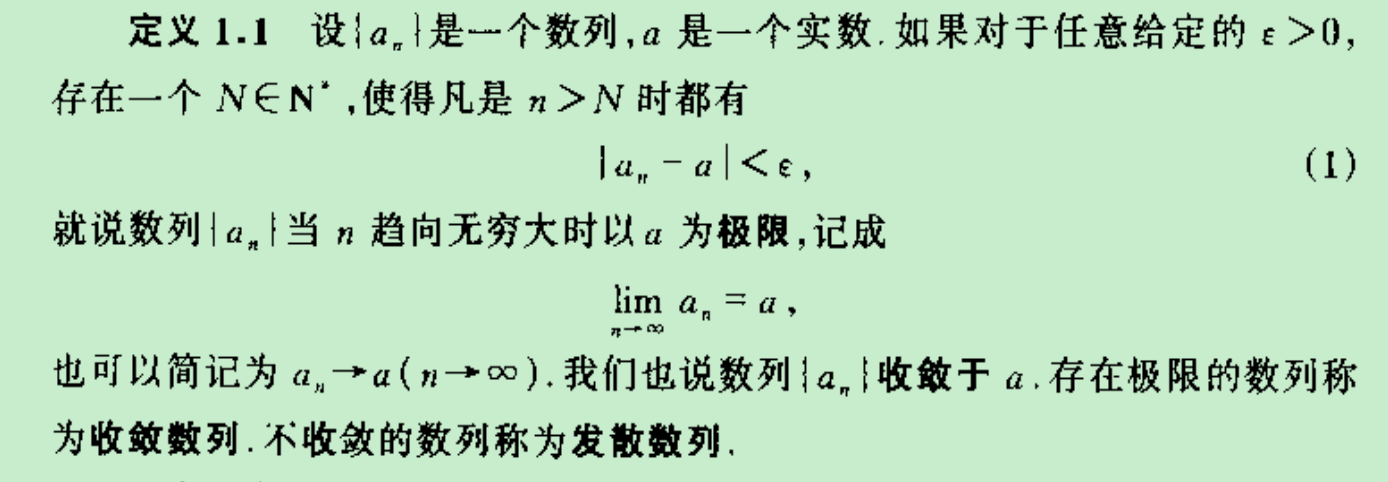1，其中我们着重来看$\lim_{n \rightarrow \infty}\mspace{2mu} a_{n} = a$，这是大多数教材通常采用的对极限现象的符号代表形式，为了进一步了解极限的性质及其计算极限就有必要建立起极限的四则运算法则。如下便是利用极限的lim符号表示形式对极限四则运算法则的表达（划红线的原因请看这里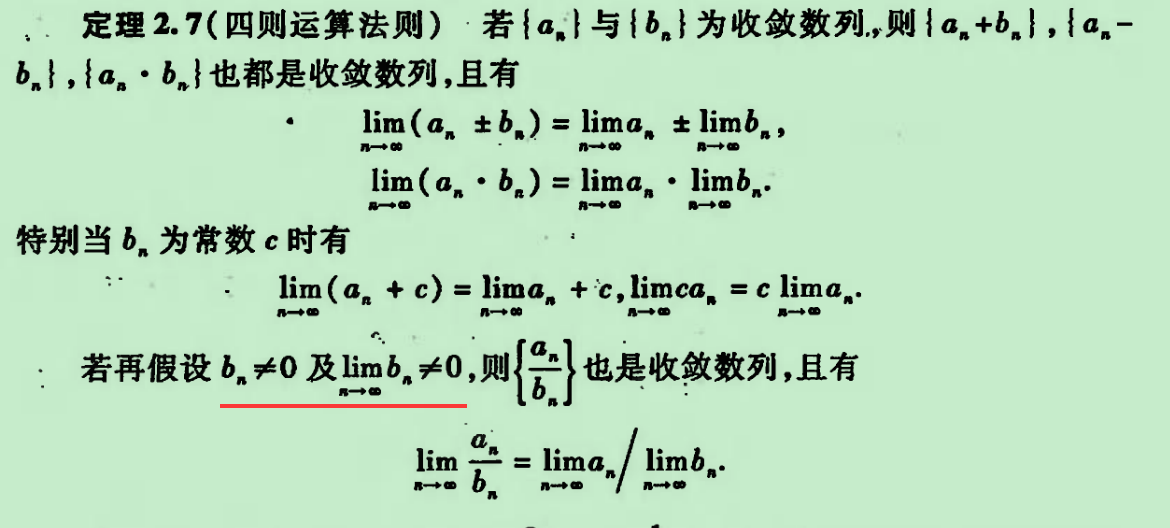2，因为这种符号代表形式难以提供什么证明上的便利，所以还得回到基本定义上来证明这些运算法则，但是我目前看到的证明都很复杂，尤其是如下所示的2和3的证明，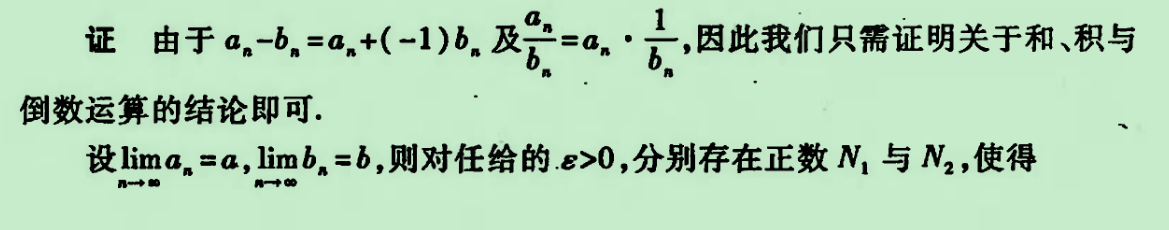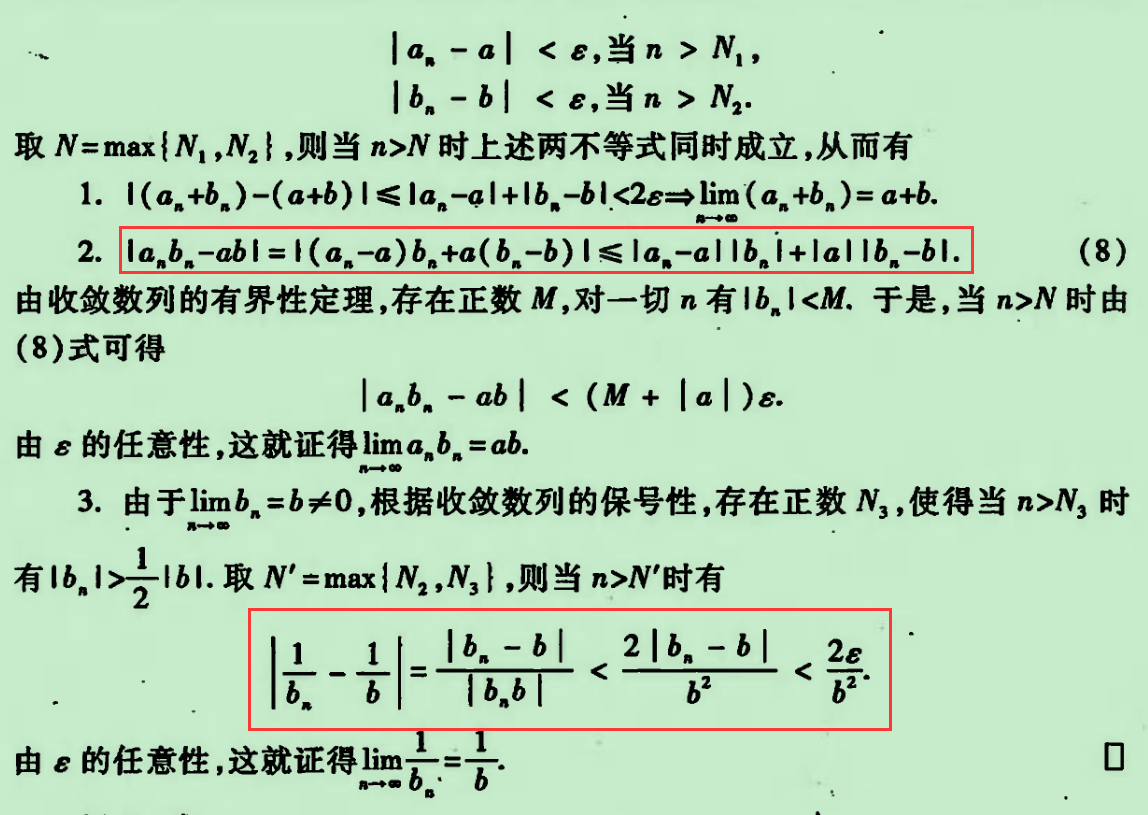3，面对这些证明，我所认为的难处在于里面的等式变形及其不等式放缩，不容易看清楚作那些变形的方向是什么——为什么会想到那些变形？所以我只能将这些变换算看作是奇思妙想，说得不好听一点则曰之“奇技淫巧”， 私以为好的数学证明应当避免这些奇技淫巧，多遵循基本技巧和方法，若总是依仗一些奇思妙想难免让人对数学望而却步，读者也难以师法其中。如下便是我对可以理解掌握的、能从中吸取到有用经验的证明方法的寻求记录。

$$\left| \frac{1}{b_{n}} - \frac{1}{b} \right| = \left| \frac{b - b_{n}}{b_{n}b} \right|$$

$$\left| \frac{1}{b_{n}} - \frac{1}{b} \right| = \left| \frac{b - b_{n}}{b_{n}b} \right| = \frac{|b - b_{n}|}{|b_{n}| \times |b|} < \frac{\varepsilon_{2}}{|b_{n}| \times |b|}$$

$$\left| \frac{1}{b_{n}} - \frac{1}{b} \right| = \left| \frac{b - b_{n}}{b_{n}b} \right| = \frac{|b - b_{n}|}{|b_{n}| \times |b|} < \frac{\varepsilon_{4}}{|b_{n}| \times |b|} < \frac{\varepsilon_{4}}{(|b| - \varepsilon_{4}) \times |b|}$$4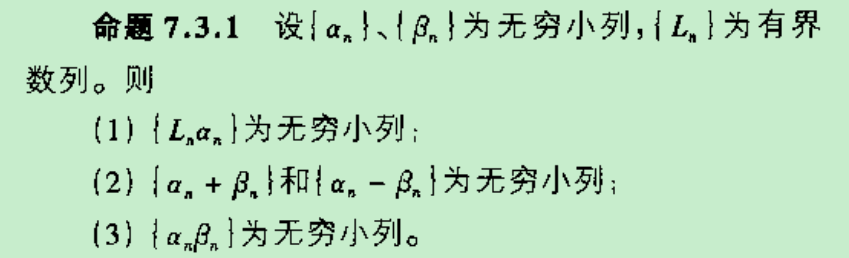5，然后再给出无穷大数列的定义：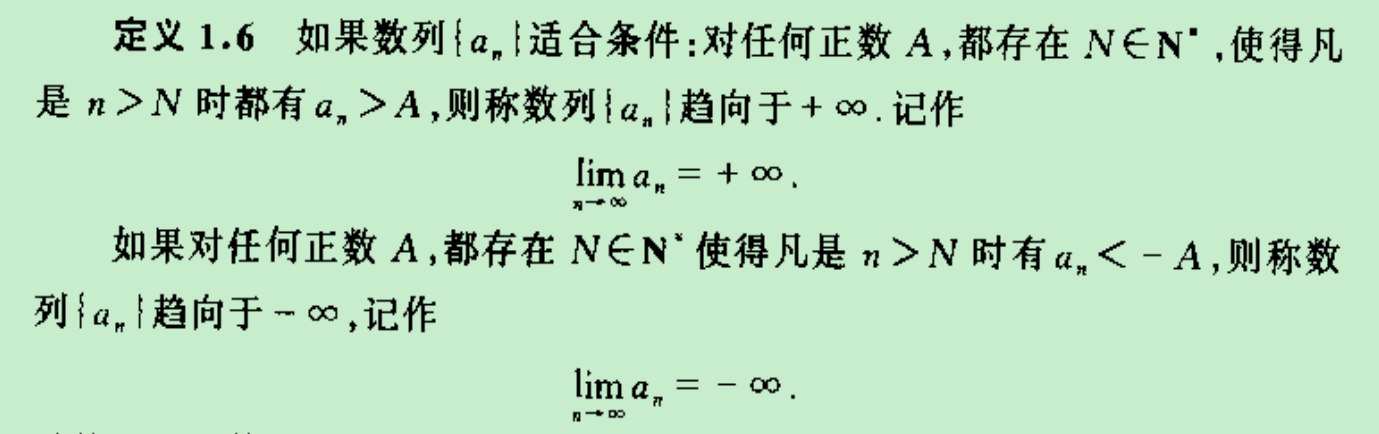6，在此基础上再证明无穷大数列相关的性质，如：（1）无穷大数列的倒数是无穷小数列；（2）无穷大数列各项加上常数后的新数列仍然是无穷大数列。建立起这些定义及其性质之后就可以提出极限定义的另外一种表达形式7，不妨称其为基于无穷小数列的数列极限定义方式：对于数列 $\left\{ a_{n} \right\}$ ，如果有实数 $a$ 和无穷小列 $\left\{ \alpha_{n} \right\}$, 使得

$$a_{n} = a + \alpha_{n},$$

$$\lim_{n \rightarrow \infty}\mspace{2mu}\left( a_{n} \cdot b_{n} \right) = \lim_{n \rightarrow \infty}\mspace{2mu}\left( (a + \alpha_{n}) \cdot (b + \beta_{n}) \right) = \lim_{n \rightarrow \infty}\mspace{2mu}\left( ab + a\beta_{n} + b\alpha_{n} + {\alpha_{n}\beta}_{n} \right)\mspace{2mu}$$

$$\lim_{n \rightarrow \infty}\mspace{2mu}\left( ab + a\beta_{n} + b\alpha_{n} + {\alpha_{n}\beta}_{n} \right) = ab$$

$$\lim_{n \rightarrow \infty}\mspace{2mu}\left( a_{n} \cdot b_{n} \right) = ab = \lim_{n \rightarrow \infty}\mspace{2mu} a_{n} \cdot \lim_{n \rightarrow \infty}b_{n}.$$

$$\lim_{n \rightarrow \infty}\mspace{2mu}\frac{1}{b_{n}} = \lim_{n \rightarrow \infty}\mspace{2mu}(\frac{1}{b} + \left( \frac{1}{b_{n}} - \frac{1}{b} \right)) = \lim_{n \rightarrow \infty}\mspace{2mu}(\frac{1}{b} + \left( \frac{1}{b + \beta_{n}} - \frac{1}{b} \right))$$

1. 数学分析教程，第二版，常庚哲，史济怀，p9↩︎

2. 数学分析（上册），第四版，华东师范大学数学系，p32↩︎

3. 数学分析（上册），第四版，华东师范大学数学系，p32-33↩︎

4. 从数学教育到教育数学，张景中，p185↩︎

5. 从数学教育到教育数学，张景中，p177↩︎

6. 数学分析教程，第二版，常庚哲，史济怀，p23↩︎

7. 从数学教育到教育数学，张景中，p174↩︎